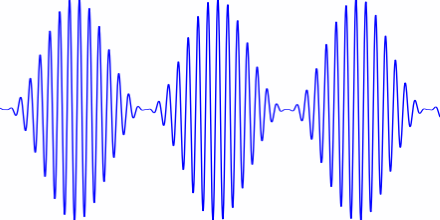# Psychoacoustic Roughness

### Sometimes audio signals are rough problems to analyze. Who knew that it was a parameter of acoustic roughness? Well there is and it's called the asper.

· Big Data Zone · Code Snippet
Save
3.39K Views

acoustic roughness is measured in aspers. an asper is the roughness of a 1 khz tone, at 60 db, 100% modulated at 70 hz. that is, the signal

(1 + sin(140π t )) sin(2000π t )

where t is time in seconds.here’s what that sounds like (if you play this at 60 db, about the loudness of a typical conversation at one meter):

and here’s the python code that made the file:

``````from scipy.io.wavfile import write
from numpy import arange, pi, sin, int16

def f(t, f_c, f_m):
# t    = time
# f_c  = carrier frequency
# f_m  = modulation frequency
return (1 + sin(2*pi*f_m*t))*sin(2*f_c*pi*t)

def to_integer(signal):
# take samples in [-1, 1] and scale to 16-bit integers,
# values between -2^15 and 2^15 - 1.
return int16(signal*(2**15 - 1))

n = 48000 # samples per second
x = arange(3*n) # three seconds of audio

# 1 asper corresponds to a 1 khz tone, 100% modulated at 70 hz, at 60 db
data = f(x/n, 1000, 70)
write("one_asper.wav", n, to_integer(data))``````
Topics:
python, audio processing, wave

Published at DZone with permission of John Cook.

Opinions expressed by DZone contributors are their own.